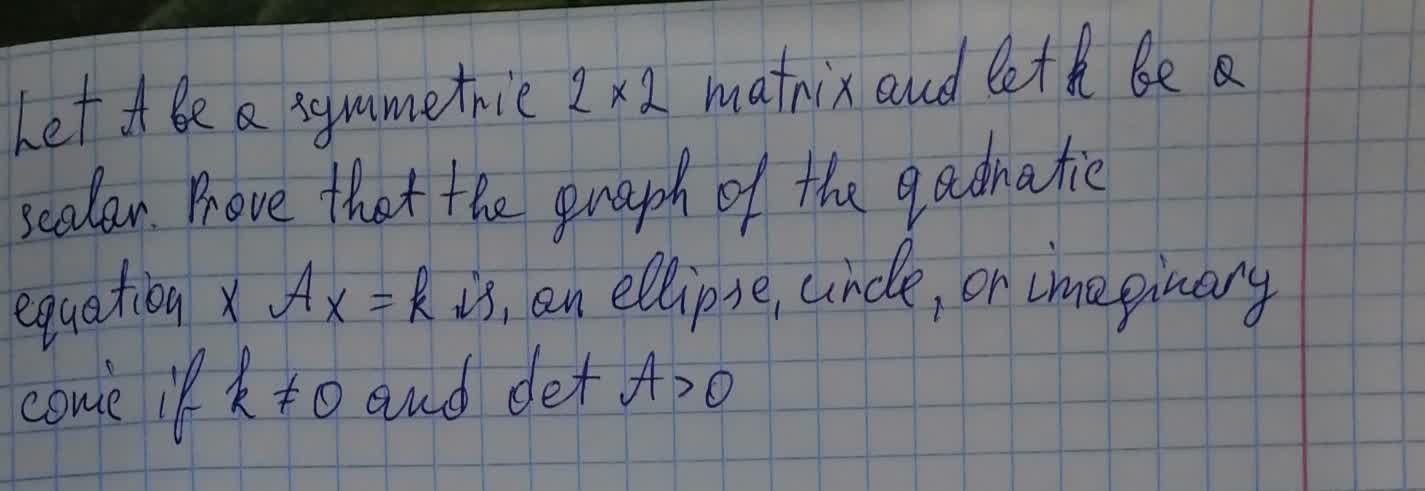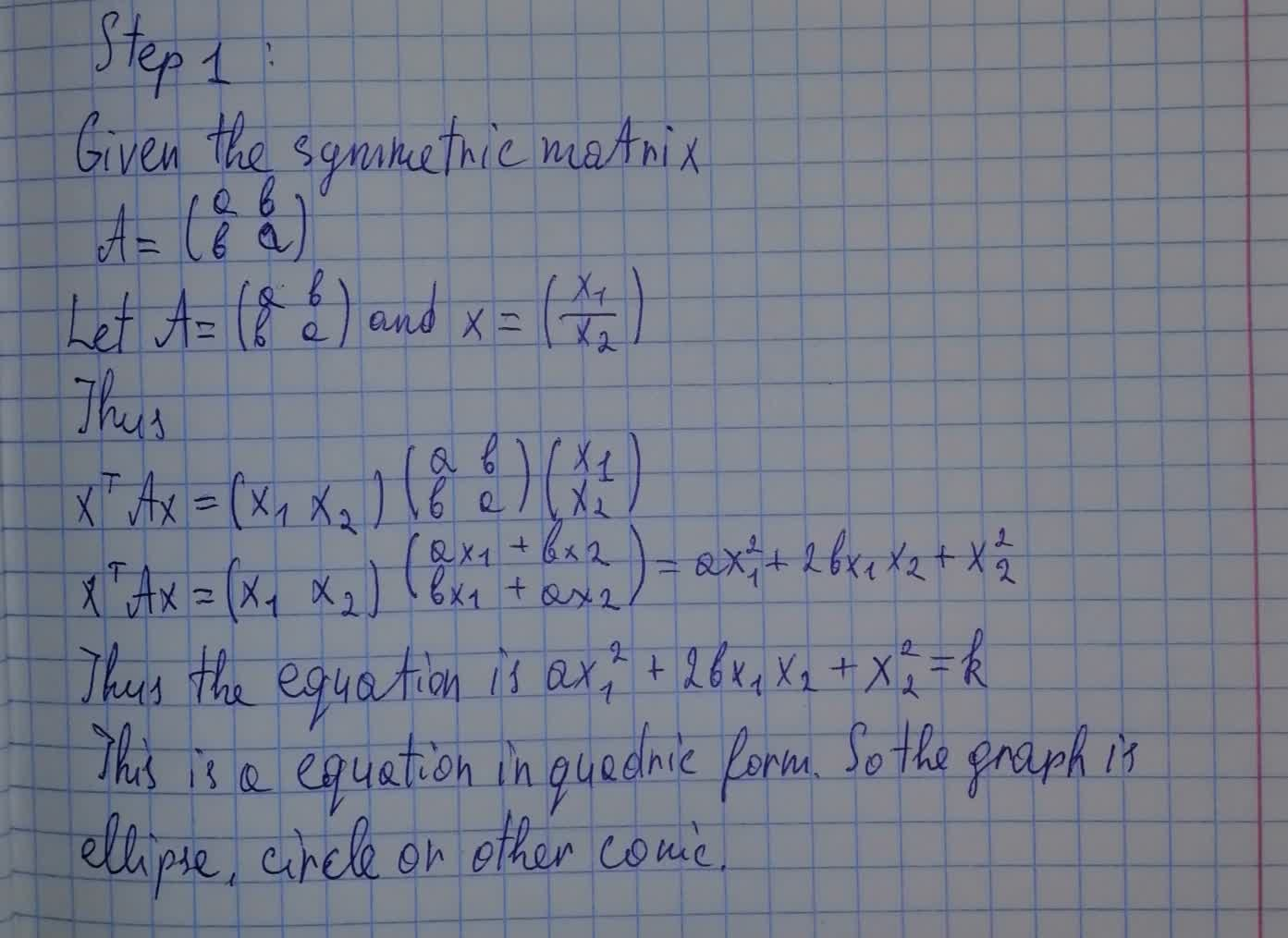Let A be a symmetric 2 \times 2 matrix and let k be a scalar. Prove that the graph of the quadratic equation x^T Ax=k is ,an ellipse, circlenitraiddQ 2021-08-10 Answered
Let A be a symmetric $$\displaystyle{2}\times{2}$$ matrix and let k be a scalar. Prove that the graph of the quadratic equation $$\displaystyle{x}^{{T}}$$ Ax=k is ,an ellipse, circle, or imaginary conic if $$\displaystyle{k}\ne{q}{0}$$ and det A > 0• Questions are typically answered in as fast as 30 minutes

Solve your problem for the price of one coffee

• Math expert for every subject
• Pay only if we can solve itRaheem Donnelly
Solution below